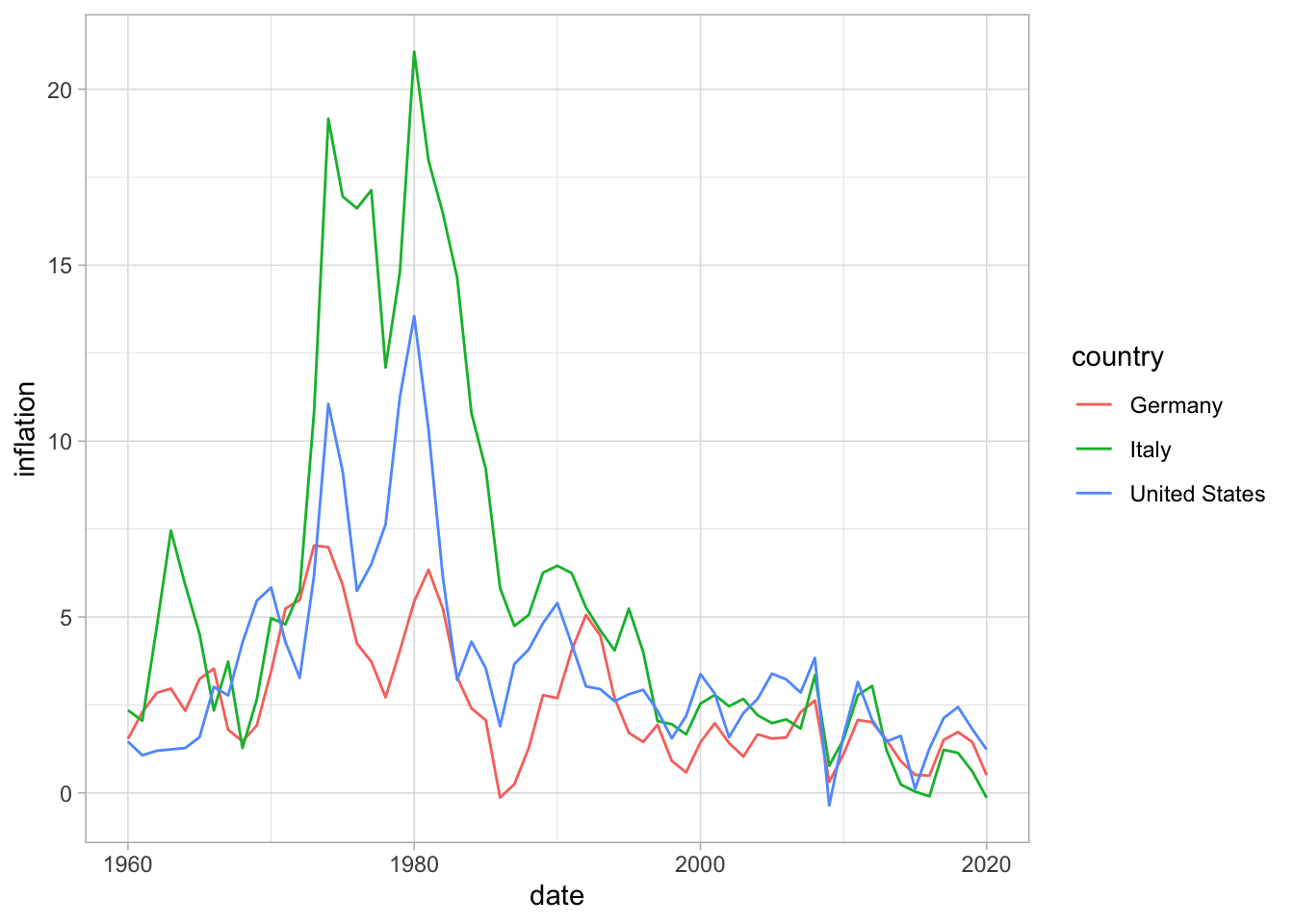# Money

## Inflation

``````wbstats::wb_search("inflation")
inflation <- wb_data("FP.CPI.TOTL.ZG", start_date = 1960, end_date = 2020)``````
``````inflation %>%
filter(country %in% c("Germany", "United States", "Italy")) %>%
select(country, date, inflation = FP.CPI.TOTL.ZG) %>%
ggplot(aes(x = date, y = inflation, col = country)) +
geom_line() +
theme_light()``````Figure 1: Inflation, consumer prices (annual %)

Previous
Next